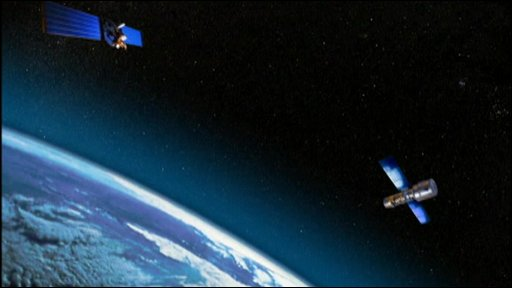Classical Mechanics

# Deriving Kepler's LawsTwo satellites each of mass $m_1 = 3.00 \text{ kg}$ and mass $m_2 = 6.00 \text{ kg}$ revolve around the same planet. The respective radii of their orbits are $r_1 = 2.00 \times 10^5 \text{ m}$ and $r_2 = 1.80 \times 10^6 \text{ m}.$ If the time period of the satellite of mass $m_1$ is $T_1 = 4.00 \times 10^5 \text{ s},$ what is that of the satellite of mass $m_2?$

Assumptions and Details

• The universal gravitational constant is $G=6.67 \times 10^{-11} \text{ N}\cdot\text{m}^2\text{/kg}^2.$

A box of mass $m = 3 \text{ kg}$ is placed on the edge of a merry-go-round of radius $r= 4 \text{ m}.$ The coefficient of static friction between the box and the merry-go-round is $\mu = 0.3 .$ What is the merry-go-round's speed squared at the moment the box slides off?

Assumptions and Details

• The gravitational acceleration is $g= 10 \text{ m/s}^2.$

A satellite of mass $m = 2.00 \times 10^3 \text{ kg}$ revolves around a planet of mass $M = 6.00 \times 10^{16} \text{ kg},$ with constant speed. If the radius of the orbit is $r = 2.00 \times 10^5 \text{ m},$ what is the satellite's approximate speed?

Assumptions and Details

• The universal gravitational constant is $G=6.67 \times 10^{-11} \text{ N}\cdot\text{m}^2\text{/kg}^2.$

A satellite of mass $m = 5.00 \times 10^3 \text{ kg}$ revolves around a planet of mass $M = 5.00 \times 10^{16} \text{ kg},$ at constant speed. If the radius of the orbit is $r = 3.00 \times 10^5 \text{ m} ,$ what is the approximate period of the revolution?

Assumptions and Details

• The universal gravitational constant is $G=6.67 \times 10^{-11} \text{ N}\cdot\text{m}^2\text{/kg}^2.$

Two identical satellites each of mass $m = 6.00 \times 10 ^3 \text{ kg}$ revolve around a planet of mass $M = 5.00 \times 10^{16} \text{ kg}.$ If the respective radii of their orbits are $r_1 = 2.00 \times 10^5 \text{ m}$ and $r_2 = 1.80 \times 10^6 \text{ m} ,$ what is the ratio between the speeds of the two satellites?

Assumptions and Details

• The universal gravitational constant is $G=6.67 \times 10^{-11} \text{ N}\cdot\text{m}^2\text{/kg}^2.$
×